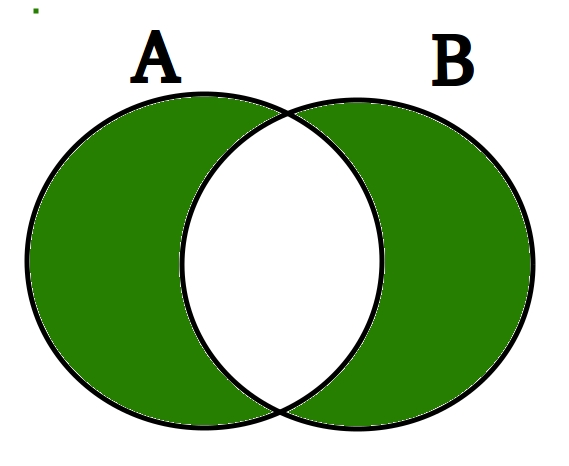Related Articles

# Python Set | symmetric_difference()

• Last Updated : 06 Feb, 2018

This in-built function of Python Set helps us to get the symmetric difference between two sets, which is equal to the elements present in either of the two sets, but not common to both the sets. Let’s look at the Venn diagram of the symmetric_difference between two sets.Symmetric Difference is marked in Green

If there are a set_A and set_B, then the symmetric difference between them will be equal to the union of set_A and set_B without the intersection between the two.

```// Takes a single parameter that has to be
// a set and returns a new set which is the
// symmetric difference between the two sets.
set_A.symmetric_difference(set_B)```

Examples:

```Input: set_A = {1, 2, 3, 4, 5}
set_B = {6, 7, 3, 9, 4}
Output :  {1, 2, 5, 6, 7, 9}
Explanation: The common elements {3, 4}

Input:
set_A = {"ram", "rahim", "ajay", "rishav", "aakash"}
set_B = {"aakash", "ajay", "shyam", "ram", "ravi"}
Output: {"rahim", "rishav", "shyam", "ravi"}

Explanation: The common elements {"ram", "ajay",
"aakash"} are discarded from the final set```

In this program we will try to find the symmetric difference between two sets:

 `# Python code to find the symmetric_difference``# Use of symmetric_difference() method`` ` `set_A ``=` `{``1``, ``2``, ``3``, ``4``, ``5``}``set_B ``=` `{``6``, ``7``, ``3``, ``9``, ``4``}``print``(set_A.symmetric_difference(set_B))`

Output:

`{1, 2, 5, 6, 7, 9}`

There is also another method to get the symmetric difference between two sets, by the use of an operator “^“.
Example:

 `# Python code to find the Symmetric difference``# using ^ operator.`` ` `# Driver Code``set_A ``=` `{``"ram"``, ``"rahim"``, ``"ajay"``, ``"rishav"``, ``"aakash"``}``set_B ``=` `{``"aakash"``, ``"ajay"``, ``"shyam"``, ``"ram"``, ``"ravi"``}``print``(set_A ^ set_B)`

Output:

`{'shyam', 'ravi', 'rahim', 'rishav'}`
 `# One more example Python code to find ``# the symmetric_difference use of ``# symmetric_difference() method`` ` `A ``=` `{``'p'``, ``'a'``, ``'w'``, ``'a'``, ``'n'``}``B ``=` `{``'r'``, ``'a'``, ``'o'``, ``'n'``, ``'e'``}``C ``=` `{}`` ` `print``(A.symmetric_difference(B))``print``(B.symmetric_difference(A))`` ` `print``(A.symmetric_difference(C))``print``(B.symmetric_difference(C))`` ` `# this example is contributed by sunny6041`

Output:

```set(['e', 'o', 'p', 'r', 'w'])
set(['e', 'o', 'p', 'r', 'w'])
set(['a', 'p', 'w', 'n'])
set(['a', 'r', 'e', 'o', 'n'])
```

Attention geek! Strengthen your foundations with the Python Programming Foundation Course and learn the basics.

To begin with, your interview preparations Enhance your Data Structures concepts with the Python DS Course. And to begin with your Machine Learning Journey, join the Machine Learning – Basic Level Course

My Personal Notes arrow_drop_up